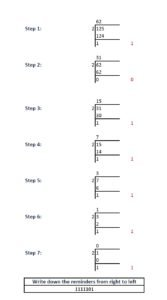Use the below conversion tool to convert a binary number to decimal number:

 Decimal Number Binary Number

Also Try:

## Decimal Number

Whole number, a decimal point and a fractional value combines to form a decimal number. The decimal point separates the whole number part from the fractional part of the number. Each digit of a decimal number can be any number from 0 to 9. Any value less than 1 is written to the right of decimal point. Decimal numbers are also known as base-10 number or counting numbers. Place value of decimal number varies as the whole number powers of 10 starting from the left of decimal point. Similarly, the place value of digits left to decimal point varies as the division of power of tens.

## Binary number

Binary numbering system uses only two symbols 0 and 1. Each digits of a binary number is referred to as bits. Binary system is also known as base -2 system. Each digit is represented by the increasing power of 2 from the LSB (Least Significant Bit). Binary system is the heart of digital electronics and is used for information flow. In digital electronics, 0 and 1 are used to denote logic states, high and low. Arithmetic operations are also possible in binary system.

## Example:Decimal number to a Binary number conversion Let us convert 125 to binary:BinaryDecimal
000101
001002
001103
010004
010105
011006
011107
100008
100109
101010
101111
110012
110113
111014
111115

Also Try: